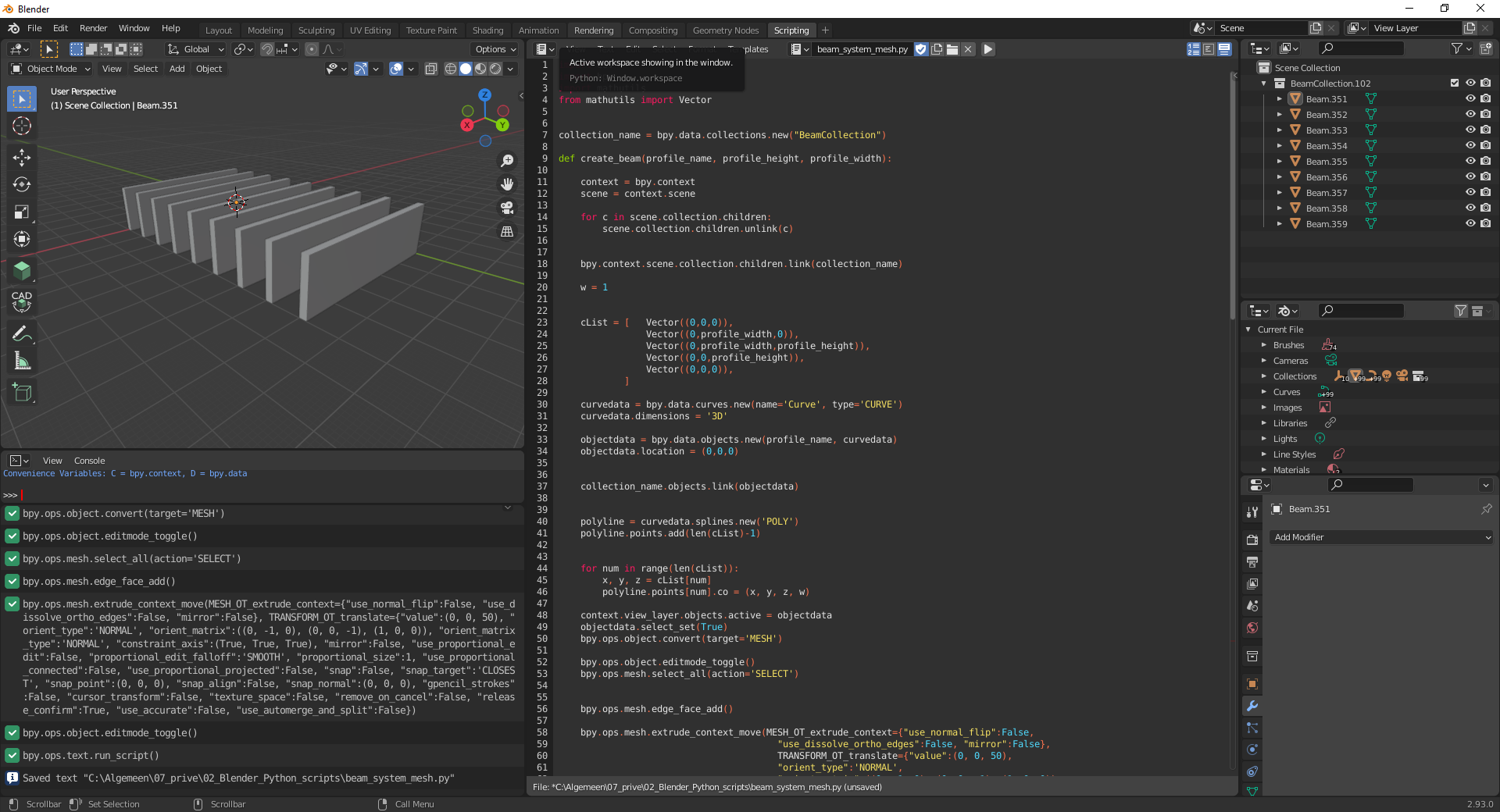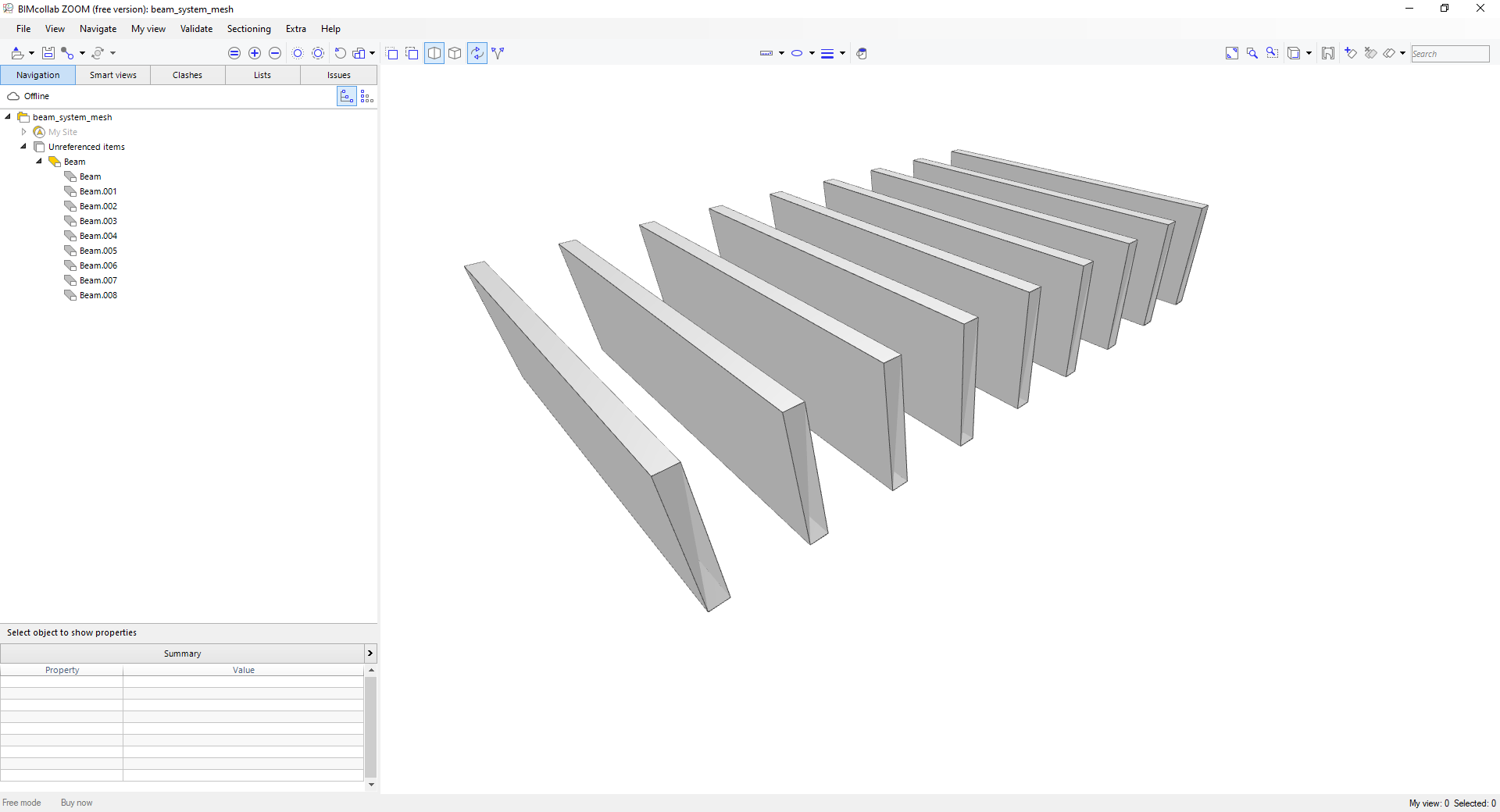Beam Systems

@Coen said:
Slightly related, are there any tools in Blender which can create a Beam plan/system just like in Revit? With span direction and boundary?

The following is the only way i know.
video: https://www.dropbox.com/s/nry2o5pqq0n5zb4/2021-09-10_11-15-10.mp4?dl=0

Unfortunately, however, IFC doesn't support arrays, as far as I know, so this would not translate into the IFC file.

• Unfortunately, however, IFC doesn't support arrays, as far as I know, so this would not translate into the IFC file.

Well, you could make an array modifier modifier in Blender, then split by objects. But for scalability it's not a good solution.

The following is the only way i know. video: https://www.dropbox.com/s/nry2o5pqq0n5zb4/2021-09-10_11-15-10.mp4?dl=0

This Boolean solution is an interesting solution, but I am thinking about ways to change the span direction too.

• I have attempted to make a very basic beam system script. The idea was I used curves to extrude along so the beam profiles are interchangeable.

import csv
import bpy
import mathutils
from mathutils import Vector

collection_name = bpy.data.collections.new("BeamCollection")

def create_profile(profile_name, profile_height, profile_width):

context = bpy.context
scene = context.scene

for c in scene.collection.children:

#collection_name = bpy.data.collections.new("BeamCollection")

w = 1

cList = [   Vector((0,0,0)),
Vector((0,profile_width,0)),
Vector((0,profile_width,profile_height)),
Vector((0,0,profile_height)),
Vector((0,0,0)),
]

curvedata = bpy.data.curves.new(name='Curve', type='CURVE')
curvedata.dimensions = '3D'

objectdata = bpy.data.objects.new(profile_name, curvedata)
objectdata.location = (0,0,0)

polyline = curvedata.splines.new('POLY')

for num in range(len(cList)):
x, y, z = cList[num]
polyline.points[num].co = (x, y, z, w)

return bpy.data.objects[objectdata.name]

def create_path(path_length):

context = bpy.context
scene = context.scene

for c in scene.collection.children:

path_name = "Path"

w = 1

cList = [   Vector((0,0,0)),
Vector((path_length,0,0)),
#Vector((0,0,0)),
]

curvedata = bpy.data.curves.new(name='Curve', type='CURVE')
curvedata.dimensions = '3D'

objectdata = bpy.data.objects.new(path_name, curvedata)
objectdata.location = (0,0,0)

polyline = curvedata.splines.new('POLY')

for num in range(len(cList)):
x, y, z = cList[num]
polyline.points[num].co = (x, y, z, w)

return bpy.data.objects[objectdata.name]

def extrude_profile_along_path(profile, path):

print (profile.name)

context = bpy.context
scene = context.scene

#sets active objects
bpy.context.view_layer.objects.active = profile

#selects active object
#profile.select_set(True)

bpy.context.object.data.bevel_mode = 'OBJECT'

bpy.context.object.data.bevel_object = bpy.data.objects[path.name]
bpy.context.object.data.use_fill_caps = True

x = 0.0
y = 0.0
z = 0.0

for i in range(0, 10):
y += 4
make_array(object=profile, x=x, y=y, z=z)

def make_array(object, x, y, z):

C = bpy.context

new_object = object
new_obj = new_object.copy()
new_obj.animation_data_clear()

# one blender unit in x-direction
vec = mathutils.Vector((x, y, z))
inv = new_obj.matrix_world.copy()
inv.invert()

# vector aligned to local axis in Blender 2.8+
vec_rot = vec @ inv
new_obj.location = new_obj.location + vec_rot

extrude_profile_along_path(profile=create_profile(profile_name="Beam",profile_height=2, profile_width=1),
path=create_path(path_length=5))

I deliberately tried to avoid using the Array Modifier because I wanted be able to translate it directly to IFC .

Next step is thinking on how to let the user create a boundary line .

I want to use this boundary line to create a an Void Box to be able to cut the beams with a Boolean tool like in the example @theoryshaw provided.

Here is the result• edited September 2021

Here a .gif demo of what I am trying to achieve.https://github.com/C-Claus/02_Blender_Python_scripts/blob/master/beam_system.py

NOTE: I see I made a mistake, the center to center distance, is not center to center, but geometry point to geometry point and I set the geometry point on the corner of the beam.

• edited September 2021

Disregard everything I posted above, different approach, I converted curves to meshed and extrude them..

import csv
import bpy
import mathutils
from mathutils import Vector

collection_name = bpy.data.collections.new("BeamCollection")

def create_beam(profile_name, profile_height, profile_width, beam_length, direction):

context = bpy.context
scene = context.scene

for c in scene.collection.children:

if c.name[0:(len(c.name)-4)] in collection_name.name:

w = 1

cList = [   Vector((0,0,0)),
Vector((0,profile_width,0)),
Vector((0,profile_width,profile_height)),
Vector((0,0,profile_height)),
Vector((0,0,0)),
]

curvedata = bpy.data.curves.new(name='Curve', type='CURVE')
curvedata.dimensions = '3D'

objectdata = bpy.data.objects.new(profile_name, curvedata)
objectdata.location = (0,0,0)

polyline = curvedata.splines.new('POLY')

for num in range(len(cList)):
x, y, z = cList[num]
polyline.points[num].co = (x, y, z, w)

context.view_layer.objects.active = objectdata

objectdata.select_set(True)
bpy.ops.object.convert(target='MESH')

bpy.ops.object.editmode_toggle()
bpy.ops.mesh.select_all(action='SELECT')

bpy.ops.mesh.extrude_context_move(MESH_OT_extrude_context={"use_normal_flip":False,
"use_dissolve_ortho_edges":False, "mirror":False},
TRANSFORM_OT_translate={"value":(0, 0, beam_length),
"orient_type":'NORMAL',
"orient_matrix":((0, -1, 0), (0, 0, -1), (1, 0, 0)),
"orient_matrix_type":'NORMAL',
"constraint_axis":(False, False, True),
"mirror":False,
"use_proportional_edit":False,
"proportional_edit_falloff":'SMOOTH',
"proportional_size":1,
"use_proportional_connected":False,
"use_proportional_projected":False,
"snap":False, "snap_target":'CLOSEST',
"snap_point":(0, 0, 0),
"snap_align":False,
"snap_normal":(0, 0, 0),
"gpencil_strokes":False,
"cursor_transform":False,
"texture_space":False,
"remove_on_cancel":False,
"release_confirm":True,
"use_accurate":False,
"use_automerge_and_split":False})

bpy.ops.object.mode_set(mode="OBJECT")
bpy.ops.object.origin_set(type='GEOMETRY_ORIGIN', center='MEDIAN')

if direction == "y":
bpy.ops.transform.rotate(value=1.5708, orient_axis='Z', orient_type='GLOBAL', orient_matrix=((1, 0, 0), (0, 1, 0), (0, 0, 1)), orient_matrix_type='GLOBAL', constraint_axis=(False, False, True), mirror=True, use_proportional_edit=False, proportional_edit_falloff='SMOOTH', proportional_size=1, use_proportional_connected=False, use_proportional_projected=False)

return bpy.data.objects[objectdata.name]

def create_beam_system(count, center_to_center_distance, beam):

context = bpy.context
scene = context.scene

#sets active objects
bpy.context.view_layer.objects.active = beam

x = 0.0
y = 0.0
z = 0.0

for i in range(0, count-1):
y += center_to_center_distance
make_array(object=beam, x=x, y=y, z=z)

def make_array(object, x, y, z):

C = bpy.context

new_object = object

new_obj = new_object.copy()
new_obj.animation_data_clear()

# one blender unit in x-direction
vec = mathutils.Vector((x, y, z))
inv = new_obj.matrix_world.copy()
inv.invert()

# vector aligned to local axis in Blender 2.8+
vec_rot = vec @ inv
new_obj.location = new_obj.location + vec_rot

create_beam_system( count=9,
center_to_center_distance=0.6,
beam = create_beam(profile_name="Beam",
profile_height=0.05,
profile_width=0.025,
beam_length=7,
direction="x" ))

The mesh result is easier to export to IFCThe resulthttps://github.com/C-Claus/02_Blender_Python_scripts/blob/master/beam_system_mesh.py

• Ah, so much to do. In short, a beam system is no different to any other parametrically generate object(s) like stairs, railings, facade systems, and so on. What happens is that right now, any specially generated object will be marked with a pset saying that it is generated from a particular engine. That engine will then know how to deconstruct the IFC definition(s) to and from whatever you use to generate it, whether it's arrays, or a script you've written yourself.

However, this system has not been formalised, though I have got it working in simple cases (like generating extruded areas either horizontally or vertically layered elements).

Also note that beams are best presented using an IfcMaterialProfileSet. This will allow the cardinal point to be parametric as well as the profile to be easily exchanged. Examples of this are given in the demo library file. A full profile manager is being built as well.